Constructing Perpendicular bisector

Practical Geometry
Serial order wise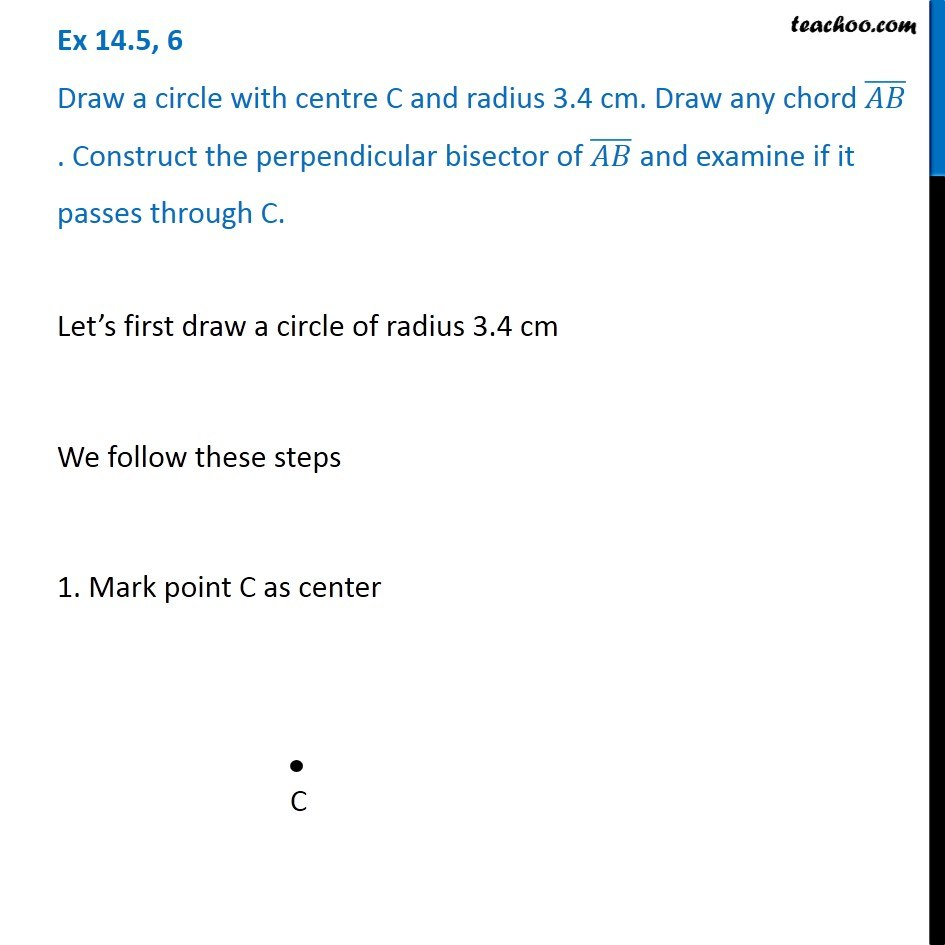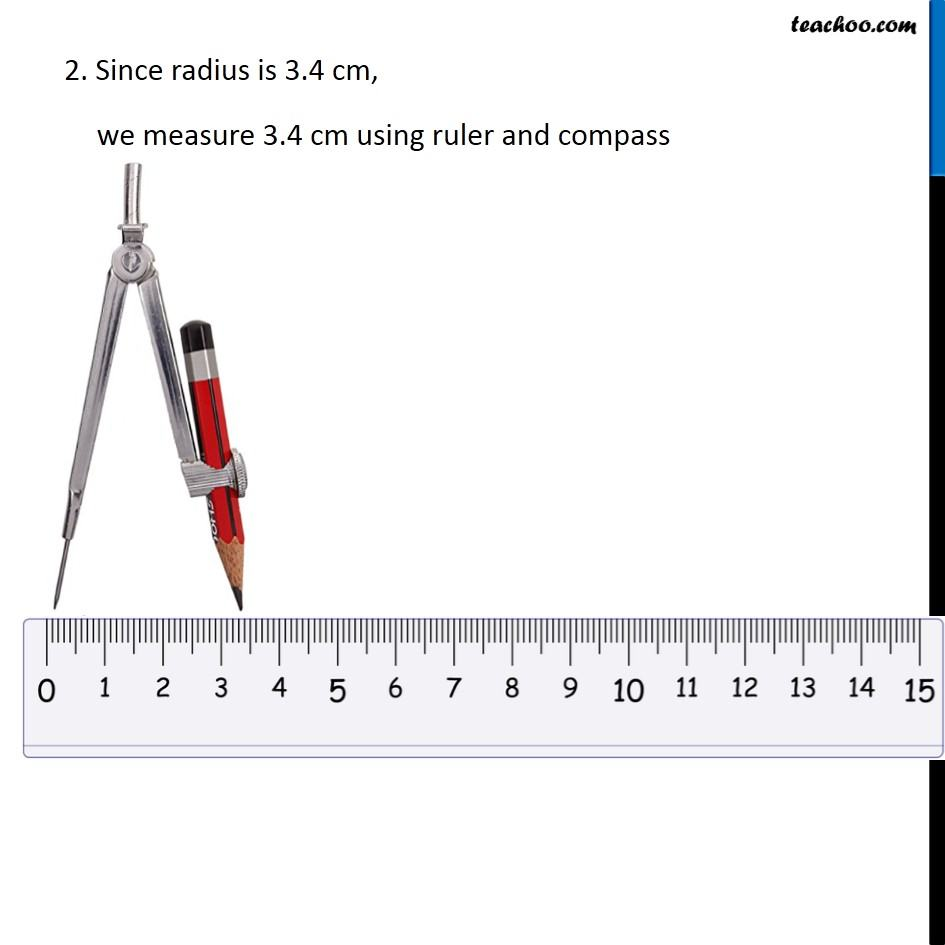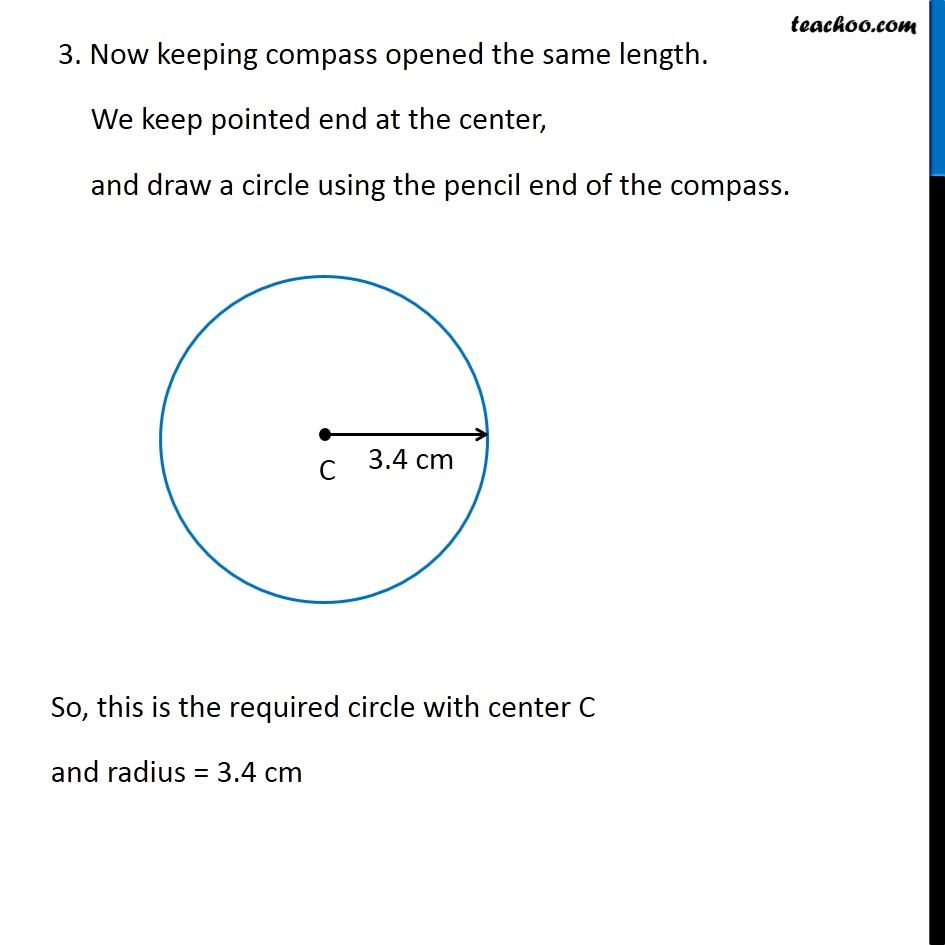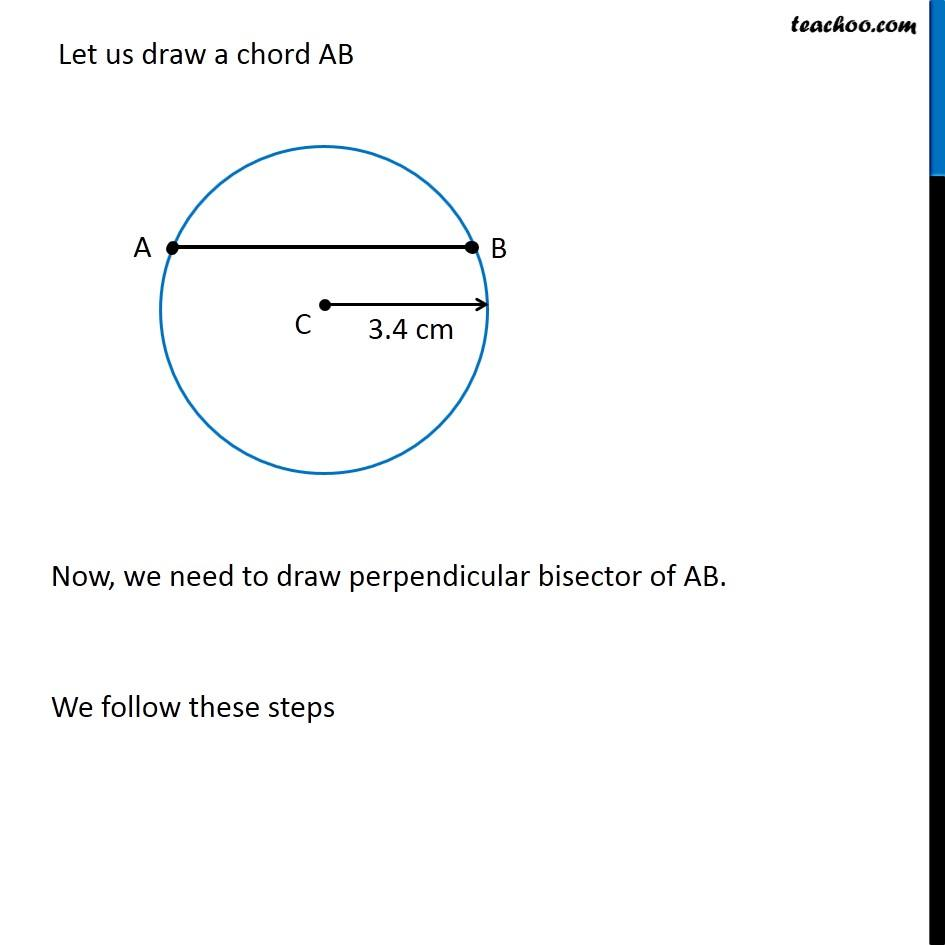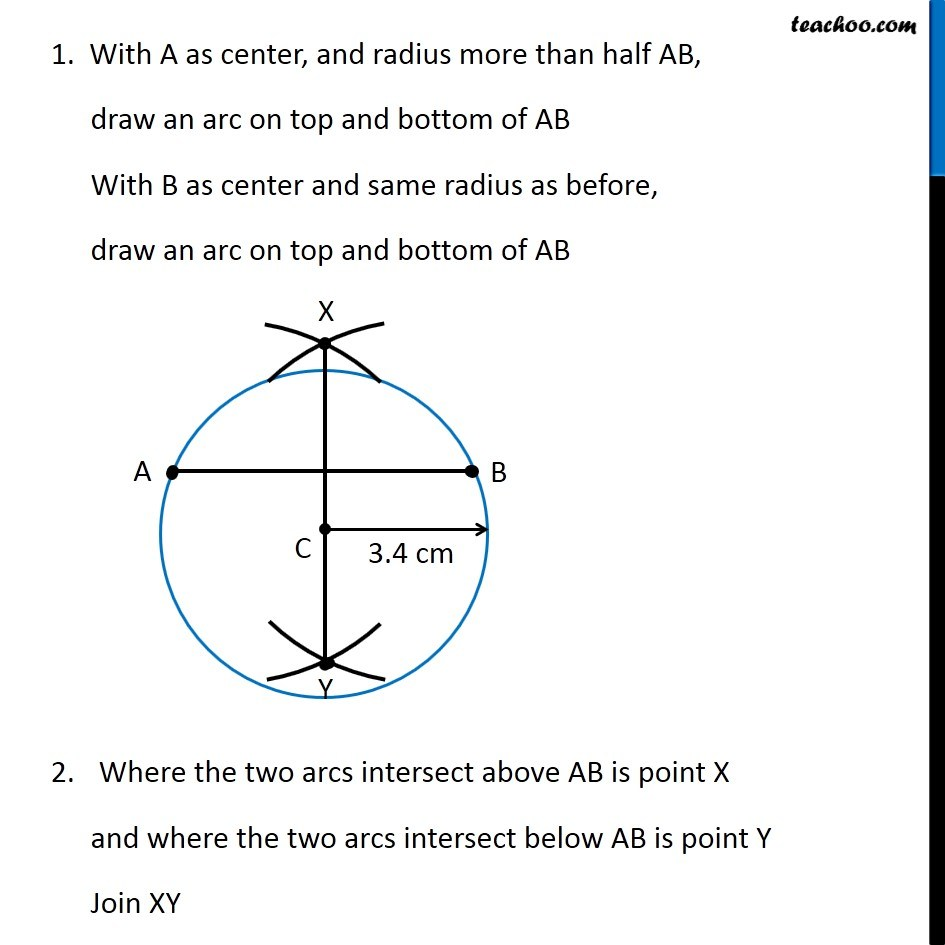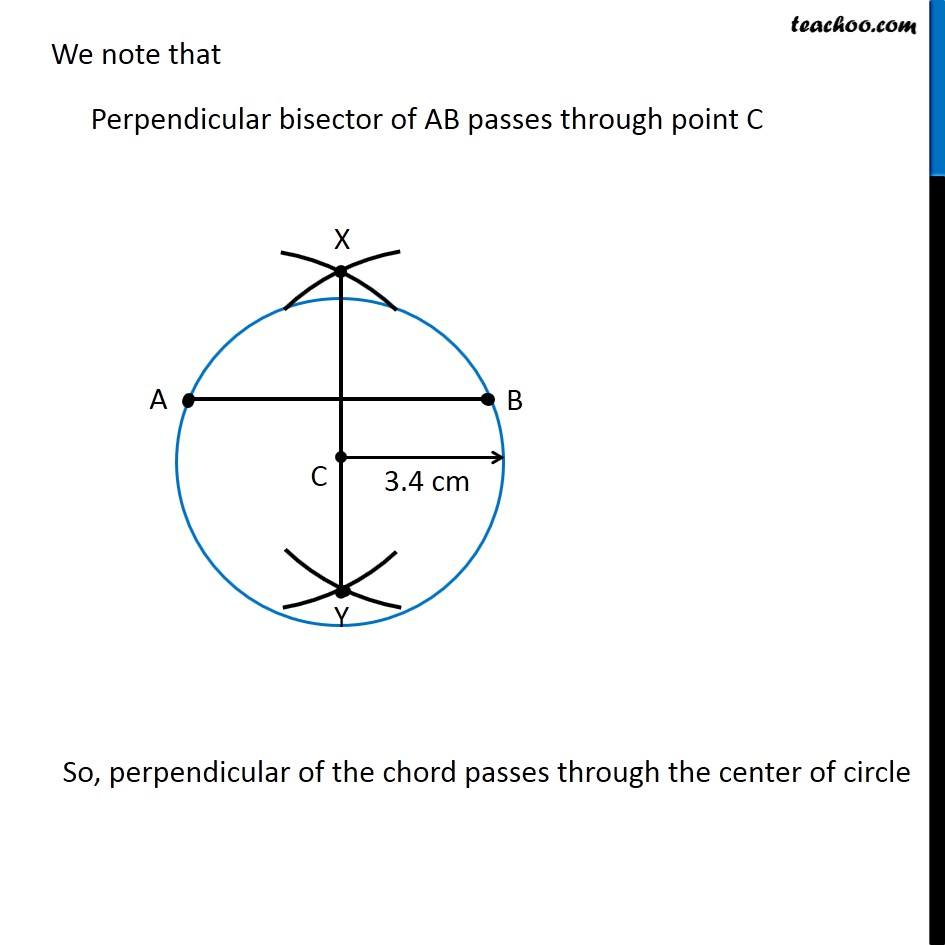Learn in your speed, with individual attention - Teachoo Maths 1-on-1 Class

### Transcript

Question 6 Draw a circle with centre C and radius 3.4 cm. Draw any chord (𝐴𝐵) ̅ . Construct the perpendicular bisector of (𝐴𝐵) ̅ and examine if it passes through C. Let’s first draw a circle of radius 3.4 cm We follow these steps 1. Mark point C as center 2. Since radius is 3.4 cm, we measure 3.4 cm using ruler and compass 3. Since radius is 3.4 cm, we measure 3.4 cm using ruler and compass So, this is the required circle with center C and radius = 3.4 cm Let us draw a chord AB Now, we need to draw perpendicular bisector of AB. We follow these steps 1. With A as center, and radius more than half AB, draw an arc on top and bottom of AB With B as center and same radius as before, draw an arc on top and bottom of AB 2.Where the two arcs intersect above AB is point X and where the two arcs intersect below AB is point Y Join XY We note that Perpendicular bisector of AB passes through point C So, perpendicular of the chord passes through the center of circle